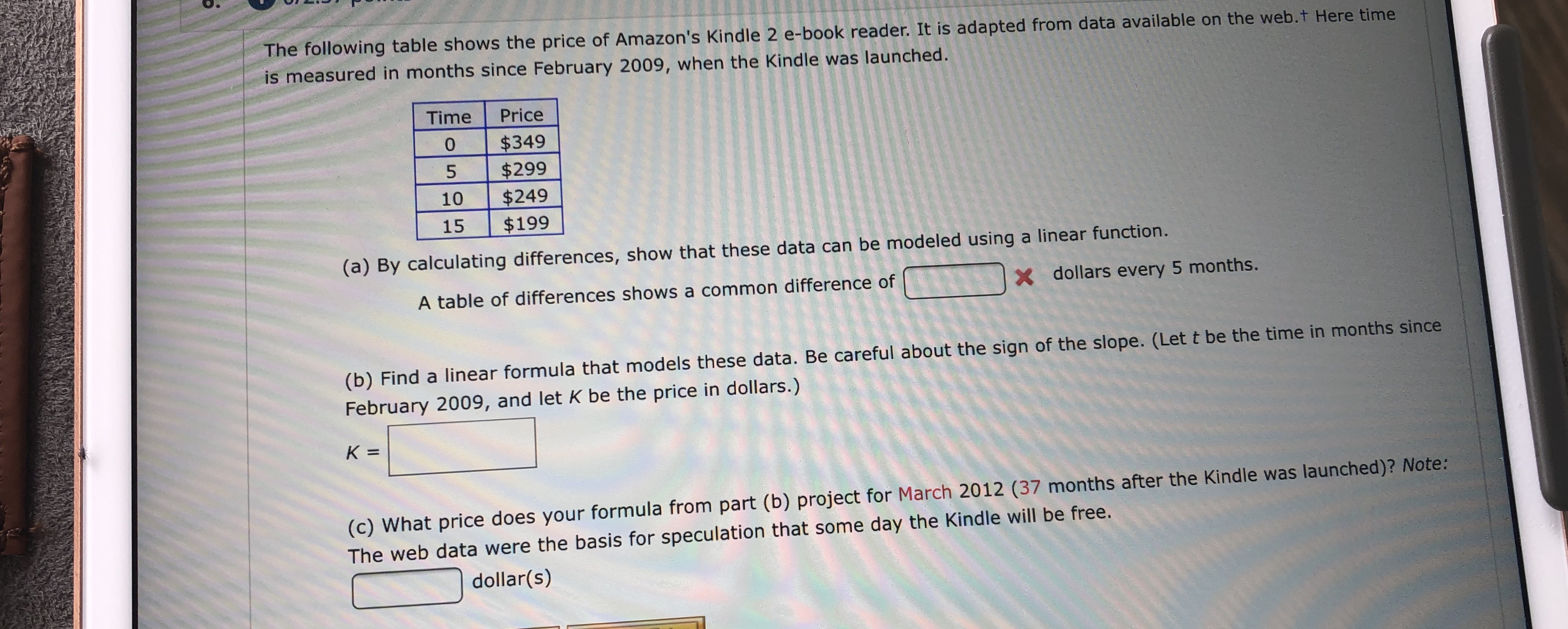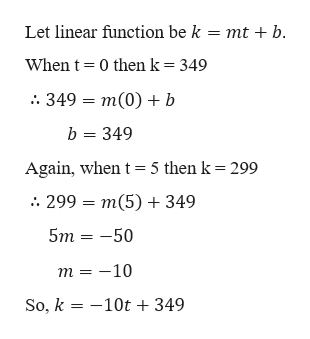# The following table shows the price of Amazon's Kindle 2 e-book reader. It is adapted from data available on the web.t Here timeis measured in months since February 2009, when the Kindle was launched.TimePrice\$349\$299\$249\$199051015(a) By calculating differences, show that these data can be modeled using a linear function.X dollars every 5 months.A table of differences shows a common difference of(b) Find a linear formula that models these data. Be careful about the sign of the slope. (Let t be the time in months sinceFebruary 2009, and let K be the price in dollars.)K =(c) What price does your formula from part (b) project for March 2012 (37 months after the Kindle was launched)? Note:The web data were the basis for speculation that some day the Kindle will be free.dollar(s)

Question
54 views

What are the blanks?help_outlineImage TranscriptioncloseThe following table shows the price of Amazon's Kindle 2 e-book reader. It is adapted from data available on the web.t Here time is measured in months since February 2009, when the Kindle was launched. Time Price \$349 \$299 \$249 \$199 0 5 10 15 (a) By calculating differences, show that these data can be modeled using a linear function. X dollars every 5 months. A table of differences shows a common difference of (b) Find a linear formula that models these data. Be careful about the sign of the slope. (Let t be the time in months since February 2009, and let K be the price in dollars.) K = (c) What price does your formula from part (b) project for March 2012 (37 months after the Kindle was launched)? Note: The web data were the basis for speculation that some day the Kindle will be free. dollar(s) fullscreen
check_circle

Step 1

Now from the given table we have

\$349 - \$ 299 = \$50

\$299 - \$ 249 = \$50

\$249 - \$ 199 = \$50

Since, the difference is constant after every interval of 5 months.

So, it can be modelled by the linear function.

Step 2

Part b

Let t be the time in months since February 20...help_outlineImage TranscriptioncloseLet linear function be k = mt + b. When t 0 then k = 349 349 = m(0)+b b 349 Again, when t 5 then k = 299 : 299 — т(5) + 349 5m 50 т %3D —10 So, k 10t +349 fullscreen

### Want to see the full answer?

See Solution

#### Want to see this answer and more?

Solutions are written by subject experts who are available 24/7. Questions are typically answered within 1 hour.*

See Solution
*Response times may vary by subject and question.
Tagged in

### Equations and In-equations